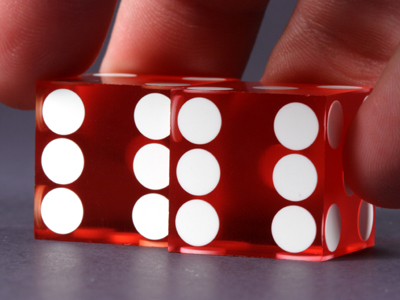6 x 6 = 36.

# The 06 Times Table

Learning the six times table is a must for any KS3 Maths student. It involves multiplying numbers by 6. There are various ways of doing this. Taking 8 x 6 as an example, we could multiply the 8 by 5 to get 40 and then add another 8 to reach 48. But that takes a little time. Learning the times tables off by heart will things a lot easier for you!

Six is a number you'll come across a lot. Insects such as flies, ants, beetles and wasps have six feet. There are six feet in a fathom. A fathom is a nautical measure of depth: it equals approximately 1.8 m. Volleyball and ice hockey are both played with teams of 6 players. A cube, also known as a hexahedron, has six faces, that's why there are six numbers on most dice.

Be careful when you pick your answers. If you rush you might hit the wrong one - and you wouldn't want that now would you? Practise makes perfect so keep coming back to this quiz even when you've mastered it.

1.
What is 10 x 6
120
80
60
30
To multiply any number by 10, all you have to do is put a zero on its end
2.
What is 1 x 6
6
3
2
1
Any number multiplied by one remains unchanged
3.
What is 8 x 6
46
44
54
48
One way to multiply a number by 8 is to double it, then double your answer and finally double it again
4.
What is 2 x 6
12
26
16
62
To multiply a number by 2 just add it to itself
5.
What is 6 x 6
36
26
42
38
36 is 6 squared, so 6 is the square root of 36
6.
What is 4 x 6
24
20
28
46
6 x 5 = 30 and 30 - 6 = 24
7.
What is 3 x 6
18
12
16
14
To work this out you could first multiply 3 x 5 and then add 3
8.
What is 9 x 6
54
56
48
58
6 x 10 = 60 and 60 - 6 = 54
9.
What is 7 x 6
46
40
42
44
In the comic sci-fi novel The Hitchhiker's Guide to the Galaxy, the answer to the ultimate question of life, the universe and everything is 42
10.
What is 5 x 6
30
36
33
25
To multiply a number by 5, first times it by 10 and then half your answer
Author:  Frank Evans